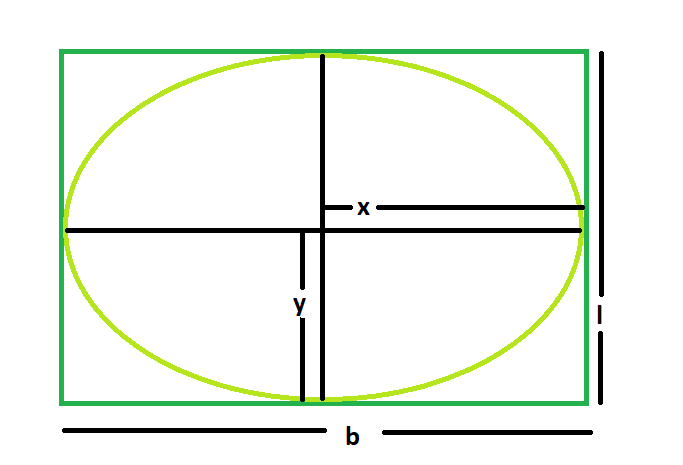# Area of the biggest ellipse inscribed within a rectangle

Given here is a rectangle of length l & breadth b, the task is to find the area of the biggest ellipse that can be inscribed within it.

Examples:

```Input: l = 5, b = 3
Output: 11.775

Input: 7, b = 4
Output: 21.98
```## Recommended: Please try your approach on {IDE} first, before moving on to the solution.

Approach:

1. Let, the length of the major axis of the ellipse = 2x and the length of the minor axis of the ellipse = 2y
2. From the diagram, it is very clear that,
2x = l
2y = b
3. So, Area of the ellipse = (π * x * y) = (π * l * b) / 4

Below is the implementation of the above approach:

## C++

 `// C++ Program to find the biggest ellipse ` `// which can be inscribed within the rectangle ` ` `  `#include ` `using` `namespace` `std; ` ` `  `// Function to find the area ` `// of the ellipse ` `float` `ellipse(``float` `l, ``float` `b) ` `{ ` ` `  `    ``// The sides cannot be negative ` `    ``if` `(l < 0 || b < 0) ` `        ``return` `-1; ` ` `  `    ``// Area of the ellipse ` `    ``float` `x = (3.14 * l * b) / 4; ` ` `  `    ``return` `x; ` `} ` ` `  `// Driver code ` `int` `main() ` `{ ` `    ``float` `l = 5, b = 3; ` `    ``cout << ellipse(l, b) << endl; ` ` `  `    ``return` `0; ` `} `

## Java

 `// Java Program to find the biggest rectangle  ` `// which can be inscribed within the ellipse  ` `import` `java.util.*;  ` `import` `java.lang.*;  ` `import` `java.io.*;  ` ` `  `class` `GFG ` `{  ` `     `  `// Function to find the area  ` `// of the rectangle  ` `static` `float` `ellipse(``float` `l, ``float` `b)  ` `{  ` `     `  `    ``// a and b cannot be negative  ` `    ``if` `(l < ``0` `|| b < ``0``)  ` `        ``return` `-``1``;  ` `    ``float` `x = (``float``)(``3.14` `* l * b) / ``4``; ` ` `  `    ``return` `x;  ` `     `  `}  ` `     `  `// Driver code  ` `public` `static` `void` `main(String args[])  ` `{  ` `    ``float` `a = ``5``, b = ``3``;  ` `    ``System.out.println(ellipse(a, b));  ` `}  ` `}  ` ` `  `// This code is contributed ` `// by Mohit Kumar `

## Python3

 `# Python3 Program to find the biggest ellipse  ` `# which can be inscribed within the rectangle  ` ` `  `# Function to find the area ` `# of the ellipse  ` `def` `ellipse(l, b):  ` ` `  `    ``# The sides cannot be negative  ` `    ``if` `l < ``0` `or` `b < ``0``:  ` `        ``return` `-``1` ` `  `    ``# Area of the ellipse  ` `    ``x ``=` `(``3.14` `*` `l ``*` `b) ``/` `4` ` `  `    ``return` `x  ` ` `  `# Driver code  ` `if` `__name__ ``=``=` `"__main__"``: ` ` `  `    ``l, b ``=` `5``, ``3` `    ``print``(ellipse(l, b))  ` ` `  `# This code is contributed  ` `# by Rituraj Jain `

## C#

 `// C# Program to find the biggest rectangle  ` `// which can be inscribed within the ellipse  ` `using` `System; ` ` `  `class` `GFG ` `{  ` `     `  `// Function to find the area  ` `// of the rectangle  ` `static` `float` `ellipse(``float` `l, ``float` `b)  ` `{  ` `     `  `    ``// a and b cannot be negative  ` `    ``if` `(l < 0 || b < 0)  ` `        ``return` `-1;  ` `    ``float` `x = (``float``)(3.14 * l * b) / 4; ` ` `  `    ``return` `x;  ` `     `  `}  ` `     `  `// Driver code  ` `public` `static` `void` `Main()  ` `{  ` `    ``float` `a = 5, b = 3;  ` `    ``Console.WriteLine(ellipse(a, b));  ` `}  ` `}  ` ` `  `// This code is contributed ` `// by Code_Mech. `

## PHP

 ` `

Output:

```11.775
```

Don’t stop now and take your learning to the next level. Learn all the important concepts of Data Structures and Algorithms with the help of the most trusted course: DSA Self Paced. Become industry ready at a student-friendly price.

My Personal Notes arrow_drop_upCheck out this Author's contributed articles.

If you like GeeksforGeeks and would like to contribute, you can also write an article using contribute.geeksforgeeks.org or mail your article to contribute@geeksforgeeks.org. See your article appearing on the GeeksforGeeks main page and help other Geeks.

Please Improve this article if you find anything incorrect by clicking on the "Improve Article" button below.

Article Tags :
Practice Tags :

Be the First to upvote.

Please write to us at contribute@geeksforgeeks.org to report any issue with the above content.